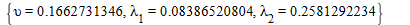6 years, 9 days

## @Carl Love  thanks for your respon...

it's quite of related to my work . depending on the shap op the list b i randomlly eliminated amember of a.

is there is any way to eliminate an amount of members of list by using asimple code in maple

## @Kitonum  thanks alot it really he...

thanks alot it really help

## @tomleslie  thanks alot sir it rea...

thanks alot sir it realy works,

i have another Question is there any tool in Sumtool help to evaluate the sum very Quiqly

## @ThU  thanks alot of your help; H...

Hfloot (infinity) it's aresult of aloop consist of 1000 iteration.

some times maple give me aresult of the integration Hfloot. I hope that enough to understand what i mean.

does the comand Expand help to make the evaluation quick over integration?

## @tomleslie  you are agenious sir i...

you are agenious sir it actually work thanks alot so much

## @mmcdara  thanks for your help sir...

thanks for your help sir i preciated it so much

## @dharr  thanks alot sir  for ...

thanks alot sir  for your help my last Question why null doesn't work with fsolve comand inside my loop

## @dharr  actually i want to look fo...

actually i want to look for the solving in +ve region and to break the loop and reapeat it with same assign of so

## @Kitonum  No that what i didnt mea...

No that what i didnt mean sir, what i ment that, i have an equation as apart of my loop and some times maple can't solve it so i was looking for acomand that  help me to test if the equation cant be solved then i assign to it another value

## @tomleslie  thanks alot sir for yo...

thanks alot sir for your help, but  fsolve comand  is not always work and help me in solving my equations . is there is any comand help me to solve my equation for example

Reylighood*distribution;
with(LinearAlgebra); with(VectorCalculus); with(Student[LinearAlgebra]); with(SignalProcessing); with(RandomTools); with(Statistics); with(stats);
f := n*R+sum((1/2)*x[g]^2, g = 1 .. n)-(sum((1/2)*(2+a[g])*x[g]^2/(1-(1-Q)*exp(-x[g]^2/(2*R))), g = 1 .. n));
f := m*S+sum((1/2)*y[t]^2, t = 1 .. m)-(sum((1/2)*(2+b[t])*y[t]^2/(1-(1-Q)*exp(-y[t]^2/(2*S))), t = 1 .. m));
f := (n+m+sum(a[g], g = 1 .. n)+sum(b[t], t = 1 .. m))/Q-(sum((2+a[g])/(exp(x[g]^2/(2*R))-1+Q), g = 1 .. n))-(sum((2+b[t])/(exp(y[t]^2/(2*S))-1+Q), t = 1 .. m));
sum(f[i]^2, i = 1 .. 3);

ggrad := Matrix([[diff(sum(f[i]^2, i = 1 .. 3), R)], [diff(sum(f[i]^2, i = 1 .. 3), S)], [diff(sum(f[i]^2, i = 1 .. 3), Q)]]);
n := 50; m := 50;
a := [seq(0, i = 1 .. 50)]; b := [seq(0, i = 1 .. 50)];

;

E1 := 0.5e-1; E2 := 0.5e-1; E3 := 0.5e-1;
K := 1000;

for so from 0 to K do W := GenerateUniform(n, 0, 1); for iii to n do vv[iii] := W[iii]^(1/(iii+sum(a[jjj], jjj = n-iii+1 .. n))) end do; for sss to n do uu[sss] := 1-product(vv[n-jjj+1], jjj = 1 .. sss); x[sss] := fsolve(1-3/(exp(t^2/(2*.6))-(1-3)) = uu[sss], t = 0 .. infinity) end do; U := GenerateUniform(m, 0, 1); for ii to m do v[ii] := U[ii]^(1/(ii+sum(b[jj], jj = m-ii+1 .. m))) end do; for ss to m do u[ss] := 1-product(v[m-jj+1], jj = 1 .. ss); y[ss] := fsolve(1-3/(exp((1/2)*t^2)-(1-3)) = u[ss], t = 0 .. infinity) end do; C := describe[quartile]([seq(x[i], i = 1 .. n)]); CC := describe[quartile]([seq(x[i], i = 1 .. n)]); CCC := describe[quartile]([seq(x[i], i = 1 .. n)]); L := describe[quartile]([seq(y[i], i = 1 .. m)]); LL := describe[quartile]([seq(y[i], i = 1 .. m)]); LLL := describe[quartile]([seq(y[i], i = 1 .. m)]); R := fsolve(3*exp(C^2/(2*R))-exp(CC^2/(2*R)) = 2, R = 0 .. infinity); S := fsolve(3*exp(L^2/(2*S))-exp(LL^2/(2*S)) = 2, S = 0 .. infinity); Q := ((eval(exp(CC^2/(2*R))-1, R = R)+eval(exp(LL^2/(2*S))-1, S = S))*(1/2)+(eval(3*exp(L^2/(2*S))-3, S = S)+eval(3*exp(C^2/(2*R))-3, R = R))*(1/2))*(1/2) end do;

## @Mariusz Iwaniuk  iam sorry if i b...

iam sorry if i bothering you; could you look please for my last  uplood wrk sheat and
help me with any suggestion?

## @Mariusz Iwaniuk thanks alot sir fo...

@Mariusz Iwaniuk
thanks alot sir for your help..........i have another Question
how can i prevent all this writting from apperence and what does this writting means ??
when i applay it into another integration i have the following massage
Error, (in evalf/int) invalid method, ftocms
here is my proplem i dealing with and unfortunately my proplem still the same>>>(1)
 >>>>>>>>(2)
 >>## @Carl Love  hi sir, iam trying to ...

hi sir, iam trying to generate asample of binomial distribution as he does, but the problem is X[i] is given as alist of one number and

x[i+1] :=x[i]-3  so x[i+1] can't be evaluated becoust it's consist of alist is there any way to transform this list to just anumber??

## @Kitonum  the problem occurs when ...

the problem occurs when i solve three equations in threes unkown parameters the result is given byand i want the first value is given to anew sympol for example called <R> and so on
is there a way to solve agroup of equations and the result  came in a list instead of agroup and could i control the naming of the results

﻿